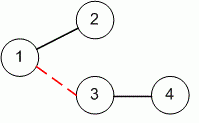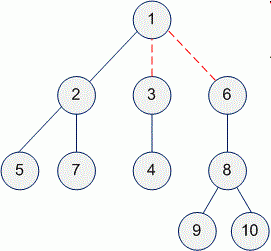Competitions

# Even Tree

Time limit 1 second
Memory limit 128 MiB

You are given a tree (a simple connected graph without cycles).

Find the maximum number of edges you can remove from the tree to get a forest such that each connected component of the forest contains an even number of nodes.

As an example, the following tree with 4 nodes can be cut at most 1 time to create an even forest.## Input data

The first line contains two integers n (2n100, n is even) and m - the number of nodes and edges. The next m lines contain two integers which specify the nodes connected by an edge of the tree. The root of the tree is node 1.

## Output data

Print the maximum number of removed edges.## Examples

Input example #1
10 9
2 1
3 1
4 3
5 2
6 1
7 2
8 6
9 8
10 8

Output example #1
2